8 years, 42 days

## MaplePrimes Activity

### These are replies submitted by maple2015

Thank you very much

Your great hints are useful for me

But I have more question (not only about lsode)

I will be grateful, if you can help me again

The value of C is assumed to be zero for relative error checking ( as you recommended)

1- It is reasonable that by assigning a little value to C, the little tolerance of C for little amount of absolute maximum step size in interval [0,1], should be ineffective to the answer. By taking the value C=Float(1,-15) and C=1E-3, the answer is  0.229491 but for C=Float(1.-14) the answer is 0.221455. Is it show the instability of solution?

2- I use the Implode and Explode commands in line 14. Is there a better way for obtaining the expression from 'RootOf' without using the String Tools?

3- Can I solve the ode in line 12 directly by maple commands?

ODE.mw

## More Questions...

Thanks a lot for your valuable hints

But I have some questions again

When we use the relative and absolute errors (C and C) simultaneously it leads to the more accuracy or using absolute error is sufficient?

Why after solution C to C  (the components of array corresponding to output data) are zero?

## @Mac Dude  Thank you for your hints...

Some of options like 'legend' and 'thickness' are available in command "curve" .

but some of options like 'label' and 'size' aren't usable in command "curve" .

IS there a way to use all options in command "curve" or plot the piecewise function

by using the command "plot" in logarithmic scale with straight lines?

Please write a code if it is possible.

## Solve.mw...

If it possible solve set of equations and only gimme amounts of P

SOLVE.mw

## .......

### But, by decreasing the number of EQs (number of EQs or nodes is arbitrary in problem), the various amounts of P can be obtained, as you can see in following attached file:

(7)Unknowns+(6)EQs.mw

###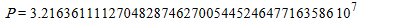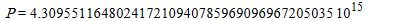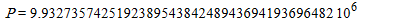## @Markiyan Hirnyk  Thank you for you...

Yes my mean is this type of coordinate

## Changinig ODE...

After changing ODE, it works

## Newton Method...

In some cases using m>6 for acceptable answer is unavoidable. But solve command don't work for m>6 !

Sol := solve(`~`[numer](Eqns), {`\$`(b[n], n = 3 .. m)}):

min(remove(has, map2(eval, F, {Sol}), I));

-------------------------------------------------------------------------------------------------------------------------------

restart: T := time(): Digits := 60:

R := sqrt(z): BI := 1.0: m := 7: g := 0.3: nu := 0.2: a := 1:b := 1.0:

w := unapply(add(b[n]*cos(1.819*n*r), n = 1 .. m), r):

W := simplify(eval(w(r), solve((D(w))(1), {b}))):

d1 := diff(W, r): d2 := diff(d1, r):

F := (int(((d2+d1/r)^2-(2*(1-nu))*d2*d1/r)*r*(1+g*r/a)^3, r = 0 .. a))/(int(d1^2*r, r = 0 .. a)):

Eqns := {seq(diff(F, b[n]), n = 3 .. m)}:

for z to m-2 do B[z, 1] := evalf(R)*BI end do:

to 30 do

for k to m-2 do for z from 3 to m do j[k, z-2] := subs({`\$`(b[n] = B[n-2, 1], n = 3 .. m)}, diff(op(`~`[numer](Eqns))[k], b[z])) end do:end do:

for k to m-2 do f[k, 1] := subs(`\$`(b[n] = B[n-2, 1], n = 3 .. m), op(`~`[numer](Eqns))[k]) end do:

FF := Matrix(m-2, 1, f):jacobi := Matrix(m-2, m-2, j):

B := evalm(Matrix(m-2, 1, B)-evalm(`&*`(1/jacobi, FF))) end do:

Error = FF; # Error of Previous Step

FW=subs({`\$`(b[n] = B[n-2, 1], n = 3 .. m)}, F);

Time = time()-T;

-------------------------------------------------------------------------------------------------------------------------------

I write above code but it is dependent to initial values of b to b[m] (i.e. BI) and ratio of b[n] (i.e. R)

For some cases Error is acceptable but the solution dosent approach to minimum amount of FW.

e.g. : (m=7, BI= 0.2,R=1, FW=157.5572)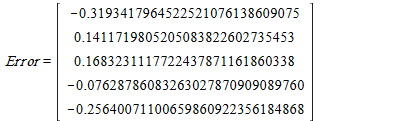e.g. : (m=7, BI= -0.9, R=sqrt(z),FW=272.8171)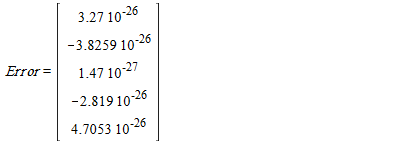For R=1/z and BI=0.05 we can get minimum amount of FW (i.e. 23.691).

But answers are changed. How can I fix it?

## Optimum Function...

It is an arbitrary selection of coefficients b[n] for satisfying bellow boundary condition, but r=1 is requisited.

`diff(w, r\$2) + nu*diff(w,r) = 0    @    r = 1`

Selection of polynomial instead of trigonometric function shows better convergence and less time for this case.

e.g.:

w1 = b[n]*r^(2+.262*n)  and w2 = b[n]*cos(n*r)  for  B.C.  " diff(w, r\$2)+nu*diff(w,r)=0 @ r=1 " :

m=3  -->  FW1=6.1123   and  FW2=6.1186    (FW1<FW2)

m=4  -->  FW1=6.1118   and  FW2=6.1123    (FW1<FW2)

m=5  -->  FW1=6.1107   and  FW2=6.1112    (FW1<FW2)

#### Exact Solution is 6.110

Cosine function is better than exponential for bellow case.

w1 = b[n]*r^(2+.506*n)  and w2 = b[n]*cos(1.8*n*r)  for  B.C.  " diff(w,r) = 0 @ r=1 " :

m=3  -->  FW1=23.7358   and  FW2=23.7796     (FW1<FW2)

m=4  -->  FW1=23.7172   and  FW2=23.7027     (FW1>FW2)

m=5  -->  FW1=23.7119   and  FW2=23.6949     (FW1>FW2)

#### Exact Solution is 23.691

It is requisted to use at least m=6 for obtaining the amount 23.691. But if it is possible, please help me to write a program for finding an optimum argument of cosine or exponent of polynomial. It may be complicated but if you have enough time please hint me about that.

Thanks

## Thanks...

Thank you tomleslie for your help

## Time Consuming...

The minimum possible amount of FW is required

Maple 18 needs unreasonable time for solving problem when m is equal to 5

Is there a way to neglect redundant equations for minimizing FW?

Assuming polynomial instead of cosine helps to convergence:

restart;

Digits := 30: m := 4: g := .3: nu := .2: a := 1:

w := unapply(add(b[n]*r^(2+.262*n), n = 1 .. m), r):

W := simplify(eval(w(r), solve(((D@@2)(w))(1)+nu*(D(w))(1), {b}))):

d1 := diff(W, r): d2 := diff(d1, r):

F := (int(((d2+d1/r)^2-(2*(1-nu))*d2*d1/r)*r*(1+g*r/a)^3, r = 0 .. a))/(int(d1^2*r, r = 0 .. a)):

Sol := solve({seq(diff(F, b[n]), n = 3 .. m)}, {`\$`(b[n], n = 3 .. m)}):

FW := min(map2(eval, F, [Sol]));

## Is it requisite to write a program for solving NL Eqs?

Tricks for solving (lots of) coupled nonlinear equations numerically

http://mathematica.stackexchange.com/questions/14824/tricks-for-solving-lots-of-coupled-nonlinear-equations-numerically

## Methods for Systems of Nonlinear Equations

http://web.engr.illinois.edu/~heath/scicomp/notes/chap05.pdf

## @tomleslie  For m>=4, Maple 18 c...

For m>=4, Maple 18 can't show numerical amounts of FW .

Maple 18 returns F parameyrically instead of FW.

m=2 ---> FW=6.18788052788535979489838829491

m=3 ---> FW=6.11860004299491357506825515146

m=4 ---> FW =

(26.6017792231159512532947857770*b^2+361.474932298954936166628403879*b^2+

294.705660784037569029349281506*b^2+176.452707059743221717141668429*b*b+

179.764515894940777468085569871*b*b+618.168221185375981314638994801*b*b)

/(4.29901306323488716726538280258*b^2+54.7320010955844337488514131001*b^2+

48.1304162916072256887555907820*b^2+28.7560435225384216726518943871*b*b+

30.3323036366089904837274461709*b*b+101.906642969089067491841606911*b*b)

The exact solution is 6.110

﻿